### Discrete Math OverviewDiscrete math is the study of mathematical structures that are fundamentally discrete as opposed to continuous. It has been characterized as the branch of mathematics dealing with countable sets. Topics in discrete math include game theory, set theory, logic, probability, and number theory, among others.

#### Discrete Math in the Classroom

Discrete math is generally encountered in advanced courses in a bachelors, masters, or doctorate math program.

#### Discrete Math Tutoring Program

Parliament's Discrete Math Tutoring Program equips students with...

• The skills necessary to master the concepts in Discrete Math.
• Effective study skills which can be translated into use for other disciplines.
• Our Discrete Math Tutors will introduce students to all the relevant fundamentals as well as important concepts to master these sections.

#### Discrete Math Tutoring Enrollment & Contact Information:

To learn more speak to an Academic Advisor, submit an Inquiry Form or use our registration forms to enroll online and meet a Discrete Math Tutor today.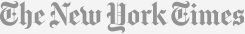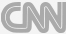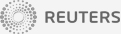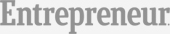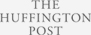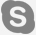#### Quick Contact

I Agree to the Terms and Conditions